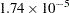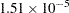## Example 65.1 Precise Analysis of Variance

The data for the following example are from Powell, Murphy, and Gramlich (1982). In order to calibrate an instrument for measuring atomic weight, 24 replicate measurements of the atomic weight of silver (chemical symbol Ag) are made with the new instrument and with a reference instrument.

Note: The results from this example vary from machine to machine, depending on floating-point configuration.

The following statements read the measurements for the two instruments into the SAS data set AgWeight:

```title 'Atomic Weight of Silver by Two Different Instruments';
data AgWeight;
input Instrument AgWeight @@;
datalines;
1 107.8681568   1 107.8681465   1 107.8681572   1 107.8681785
1 107.8681446   1 107.8681903   1 107.8681526   1 107.8681494
1 107.8681616   1 107.8681587   1 107.8681519   1 107.8681486
1 107.8681419   1 107.8681569   1 107.8681508   1 107.8681672
1 107.8681385   1 107.8681518   1 107.8681662   1 107.8681424
1 107.8681360   1 107.8681333   1 107.8681610   1 107.8681477
2 107.8681079   2 107.8681344   2 107.8681513   2 107.8681197
2 107.8681604   2 107.8681385   2 107.8681642   2 107.8681365
2 107.8681151   2 107.8681082   2 107.8681517   2 107.8681448
2 107.8681198   2 107.8681482   2 107.8681334   2 107.8681609
2 107.8681101   2 107.8681512   2 107.8681469   2 107.8681360
2 107.8681254   2 107.8681261   2 107.8681450   2 107.8681368
;
```

Notice that the variation in the atomic weight measurements is several orders of magnitude less than their mean. This is a situation that can be difficult for standard, regression-based analysis-of-variance procedures to handle correctly.

The following statements invoke the ORTHOREG procedure to perform a simple one-way analysis of variance, testing for differences between the two instruments:

```proc orthoreg data=AgWeight;
class Instrument;
model AgWeight = Instrument;
run;
```

Output 65.1.1 shows the resulting analysis.

Output 65.1.1 PROC ORTHOREG Results for Atomic Weight Example
 Atomic Weight of Silver by Two Different Instruments

The ORTHOREG Procedure

Class Level Information
Factor Levels Values
Instrument 2 1 2

 Atomic Weight of Silver by Two Different Instruments

The ORTHOREG Procedure

Dependent Variable: AgWeight

Source DF Sum of Squares Mean Square F Value Pr > F
Model 1 3.6383419E-9 3.6383419E-9 15.95 0.0002
Error 46 1.0495173E-8 2.281559E-10
Corrected Total 47 1.4133515E-8

 Root MSE 1.51048e-05 0.257427

Parameter DF Parameter Estimate Standard Error t Value Pr > |t|
Intercept 1 107.868136354166 3.0832608E-6 3.499E7 <.0001
(Instrument='1') 1 0.00001741249999 4.3603893E-6 3.99 0.0002
(Instrument='2') 0 0 . . .

The mean difference between instruments is about(the value of the (Instrument=’1’) parameter in the parameter estimates table), whereas the level of background variation in the measurements is about(the value of the root mean square error). At this level of error, the difference is significant, with a-value of 0.0002.

The National Institute of Standards and Technology (1998) has provided certified ANOVA values for this data set. The following statements use ODS to examine the ANOVA values produced by both the ORTHOREG and GLM procedures more precisely for comparison with the NIST-certified values:

```ods listing close;
ods output ANOVA         = OrthoregANOVA
FitStatistics = OrthoregFitStat;

proc orthoreg data=AgWeight;
class Instrument;
model AgWeight = Instrument;
run;

ods output OverallANOVA  = GLMANOVA
FitStatistics = GLMFitStat;
proc glm data=AgWeight;
class Instrument;
model AgWeight = Instrument;
run;
ods listing;
```
```data _null_; set OrthoregANOVA  (in=inANOVA)
OrthoregFitStat(in=inFitStat);
if (inANOVA) then do;
if (Source = 'Model') then put "Model SS: " ss e20.;
if (Source = 'Error') then put "Error SS: " ss e20.;
end;
if (inFitStat) then do;
if (Statistic = 'Root MSE') then
put "Root MSE: " nValue1 e20.;
if (Statistic = 'R-Square') then
put "R-Square: " nValue1 best20.;
end;
data _null_; set GLMANOVA  (in=inANOVA)
GLMFitStat(in=inFitStat);
if (inANOVA) then do;
if (Source = 'Model') then put "Model SS: " ss e20.;
if (Source = 'Error') then put "Error SS: " ss e20.;
end;
if (inFitStat) then      put "Root MSE: " RootMSE e20.;
if (inFitStat) then   put "R-Square: " RSquare best20.;
run;
```

In SAS/STAT software prior to SAS 8, PROC GLM gave much less accurate results than PROC ORTHOREG. Table 65.8 and Table 65.9 compare the ANOVA values certified by NIST with those produced by the two procedures.

Table 65.8 Accuracy Comparison for Sums of Squares

Values

Model SS

Error SS

NIST-certified

3.6383418750000E09

1.0495172916667E08

ORTHOREG

3.6383418747907E09

1.0495172916797E08

GLM, since SAS 8

3.6383418747907E09

1.0495172916797E08

GLM, before SAS 8

0

1.0331496763990E08

Table 65.9 Accuracy Comparison for Fit Statistics

Values

Root MSE

R Square

NIST-certified

1.5104831444641E05

0.25742654453832

ORTHOREG

1.5104831444735E05

0.25742654452494

GLM, since SAS 8

1.5104831444735E05

0.25742654452494

GLM, before SAS 8

1.4986585859992E05

0

Although the PROC ORTHOREG values and the PROC GLM values for the current version are quite close to the certified ones, the PROC GLM values for releases prior to SAS 8 are not. In fact, since the model sum of squares is so small, in prior releases the GLM procedure set it (and consequently R square) to zero.